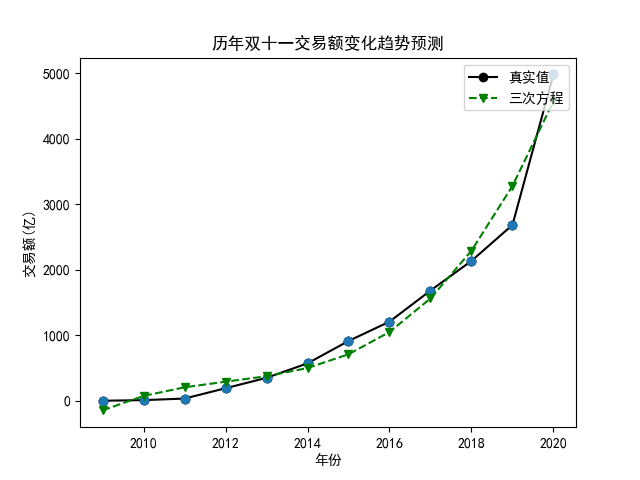# 实现过程

OK，具体是如何实现的呢？首先，我们从 CSV 文件中加载数据，这个非常简单，利用 Pandas 库中的 read_csv() 方法即可：

df = pd.read_csv('./历年双十一交易额.csv', index_col = 0)


year = np.array(df.index.tolist())
sales = np.array(df.Sales.tolist())


plt.scatter(year, sales, marker='o')
plt.xlabel('年份')
plt.ylabel('交易额(亿)')
plt.title('历年双十一交易额变化趋势')


X = (year - 2008).reshape(-1, 1)
X = np.concatenate([X], axis= -1)
Y = sales
lr = LinearRegression()
lr.fit(X, Y)
print('方程系数：', lr.coef_)
print('方程截距：',lr.intercept_)


X_train, X_test, Y_train, Y_test = train_test_split(X, Y, test_size=0.3, random_state=0)


f = lambda x: lr.coef_ * x + lr.intercept_
print('2021年交易额预测：', f(13))


print('R^2：', lr.score(X_test, Y_test))


X = (year - 2008).reshape(-1, 1)
X = np.concatenate([X**2, X], axis= -1)


X = (year - 2008).reshape(-1, 1)
X = np.concatenate([X**3, X**2, X], axis= -1)